# 8.9 Inverse Matrices

$$\newcommand{\vecs}{\overset { \rightharpoonup} {\mathbf{#1}} }$$ $$\newcommand{\vecd}{\overset{-\!-\!\rightharpoonup}{\vphantom{a}\smash {#1}}}$$$$\newcommand{\id}{\mathrm{id}}$$ $$\newcommand{\Span}{\mathrm{span}}$$ $$\newcommand{\kernel}{\mathrm{null}\,}$$ $$\newcommand{\range}{\mathrm{range}\,}$$ $$\newcommand{\RealPart}{\mathrm{Re}}$$ $$\newcommand{\ImaginaryPart}{\mathrm{Im}}$$ $$\newcommand{\Argument}{\mathrm{Arg}}$$ $$\newcommand{\norm}{\| #1 \|}$$ $$\newcommand{\inner}{\langle #1, #2 \rangle}$$ $$\newcommand{\Span}{\mathrm{span}}$$ $$\newcommand{\id}{\mathrm{id}}$$ $$\newcommand{\Span}{\mathrm{span}}$$ $$\newcommand{\kernel}{\mathrm{null}\,}$$ $$\newcommand{\range}{\mathrm{range}\,}$$ $$\newcommand{\RealPart}{\mathrm{Re}}$$ $$\newcommand{\ImaginaryPart}{\mathrm{Im}}$$ $$\newcommand{\Argument}{\mathrm{Arg}}$$ $$\newcommand{\norm}{\| #1 \|}$$ $$\newcommand{\inner}{\langle #1, #2 \rangle}$$ $$\newcommand{\Span}{\mathrm{span}}$$$$\newcommand{\AA}{\unicode[.8,0]{x212B}}$$

Two numbers are multiplicative inverses if their product is 1. Every number besides the number 0 has a multiplicative inverse. For matrices, two matrices are inverses of each other if they multiply to be the identity matrix.

What kinds of matrices do not have inverses?

Inverses of Matrices

Multiplicative inverses are two numbers or matrices whose product is one or the identity matrix. Consider a matrix $$A$$ that has inverse $$A^{-1}$$. How do you find matrix $$A^{-1}$$ if you just have matrix $$A$$ ?

A=\left[\begin{array}{ccc}

1 & 2 & 3 \\

1 & 0 & 1 \\

0 & 2 & -1

\end{array}\right], A^{-1}=?

The answer is that you augment matrix $$A$$ with the identity matrix and row reduce.

$$\left[\begin{array}{ccc|ccc}1 & 2 & 3 & 1 & 0 & 0 \\ 1 & 0 & 1 & 0 & 1 & 0 \\ 0 & 2 & -1 & 0 & 0 & 1\end{array}\right]$$

\begin{aligned} R_{1} \cdot-1+R_{2} & \rightarrow\left[\begin{array}{ccc|ccc}1 & 2 & 3 & 1 & 0 & 0 \\ 0 & -2 & -2 & -1 & 1 & 0 \\ 0 & 2 & -1 & 0 & 0 & 1\end{array}\right] \\ R_{2}+R_{3} & \rightarrow\left[\begin{array}{ccc|ccc}1 & 2 & 3 & 1 & 0 & 0 \\ 0 & -2 & -2 & -1 & 1 & 0 \\ 0 & 0 & -3 & -1 & 1 & 1\end{array}\right] \\ R_{2} \div-2 & \rightarrow\left[\begin{array}{ccc|ccc}1 & 2 & 3 & 1 & 0 & 0 \\ 0 & 1 & 1 & \frac{1}{2} & -\frac{1}{2} & 0 \\ 0 & 0 & -3 & -1 & 1 & 1\end{array}\right] \end{aligned}

$$R_{3} \div-3 \rightarrow\left[\begin{array}{ccc|ccc}1 & 2 & 3 & 1 & 0 & 0 \\ 0 & 1 & 1 & \frac{1}{2} & -\frac{1}{2} & 0 \\ 0 & 0 & 1 & \frac{1}{3} & -\frac{1}{3} & -\frac{1}{3}\end{array}\right]$$

$$R_{3} \cdot-3+R_{1} \rightarrow\left[\begin{array}{ccc|ccc}1 & 2 & 0 & 0 & 1 & 1 \\ 0 & 1 & 1 & \frac{1}{2} & -\frac{1}{2} & 0 \\ 0 & 0 & 1 & \frac{1}{3} & -\frac{1}{3} & -\frac{1}{3}\end{array}\right]$$

$$R_{3} \cdot-1+R_{2} \rightarrow\left[\begin{array}{ccc|ccc}1 & 2 & 0 & 0 & 1 & 1 \\ 0 & 1 & 0 & \frac{1}{6} & -\frac{1}{6} & \frac{1}{3} \\ 0 & 0 & 1 & \frac{1}{3} & -\frac{1}{3} & -\frac{1}{3}\end{array}\right]$$

$$R_{2} \cdot-2+R_{1} \rightarrow\left[\begin{array}{ccc|ccc}1 & 0 & 0 & -\frac{1}{3} & \frac{4}{3} & \frac{1}{3} \\ 0 & 1 & 0 & \frac{1}{6} & -\frac{1}{6} & \frac{1}{3} \\ 0 & 0 & 1 & \frac{1}{3} & -\frac{1}{3} & -\frac{1}{3}\end{array}\right]$$

The matrix on the right is the inverse matrix $$A^{-1}$$.

$$A^{-1}=\left[\begin{array}{ccc}-\frac{1}{3} & \frac{4}{3} & \frac{1}{3} \\ \frac{1}{6} & -\frac{1}{6} & \frac{1}{3} \\ \frac{1}{3} & -\frac{1}{3} & -\frac{1}{3}\end{array}\right]$$

Fractions are usually unavoidable when computing inverses.

One reason why inverses are so powerful is because they allow you to solve systems of equations with the same logic as you would solve a single linear equation. Consider the following system based on the coefficients of matrix $$A$$ from above.

\begin{aligned} x+2 y+3 z &=96 \\ x+0 y+z &=36 \\ 0 x+2 y-z &=-12 \end{aligned}

By writing this system as a matrix equation you get:

$$\left[\begin{array}{lll}1 & 2 & 3 \\ 1 & 0 & 1 \\ 0 & 2 & -1\end{array}\right] \cdot\left[\begin{array}{l}x \\ y \\ z\end{array}\right]=\left[\begin{array}{c}96 \\ 36 \\ -12\end{array}\right]$$

$$A \cdot\left[\begin{array}{l}x \\ y \\ z\end{array}\right]=\left[\begin{array}{c}96 \\ 36 \\ -12\end{array}\right]$$

If this were a normal linear equation where you had a constant times the variable equals a constant, you would multiply both sides by the multiplicative inverse of the coefficient. Do the same in this case.

$$A^{-1} \cdot A \cdot\left[\begin{array}{l}x \\ y \\ z\end{array}\right]=A^{-1} \cdot\left[\begin{array}{c}96 \\ 36 \\ -12\end{array}\right]$$

$$\left[\begin{array}{l}x \\ y \\ z\end{array}\right]=A^{-1} \cdot\left[\begin{array}{c}96 \\ 36 \\ -12\end{array}\right]$$

All that is left is for you to substitute in and to perform the matrix multiplication to get the solution.

$$\left[\begin{array}{l}x \\ y \\ z\end{array}\right]=A^{-1} \cdot\left[\begin{array}{c}96 \\ 36 \\ -12\end{array}\right]$$

$$\left[\begin{array}{l}x \\ y \\ z\end{array}\right]=\left[\begin{array}{ccc}-\frac{1}{3} & \frac{4}{3} & \frac{1}{3} \\ \frac{1}{6} & -\frac{1}{6} & \frac{1}{3} \\ \frac{1}{3} & -\frac{1}{3} & -\frac{1}{3}\end{array}\right] \cdot\left[\begin{array}{c}96 \\ 36 \\ -12\end{array}\right]$$

$$\left[\begin{array}{l}x \\ y \\ z\end{array}\right]=\left[\begin{array}{c}-\frac{1}{3} \cdot 96+\frac{4}{3} \cdot 36+\frac{1}{3} \cdot(-12) \\ \frac{1}{6} \cdot 96-\frac{1}{6} \cdot 36+\frac{1}{3} \cdot(-12) \\ \frac{1}{3} \cdot 96-\frac{1}{3} \cdot 36-\frac{1}{3} \cdot(-12)\end{array}\right]$$

$$\left[\begin{array}{l}x \\ y \\ z\end{array}\right]=\left[\begin{array}{c}12 \\ 6 \\ 24\end{array}\right]$$

## Examples

##### Example 1

Earlier, you were asked what types of matrices do not have inverses. Non-square matrices do not generally have inverses. Square matrices that have determinants equal to zero do not have inverses.

##### Example 2

Find the inverse of the following matrix.

$$\left[\begin{array}{cc}1 & 6 \\ 4 & 24\end{array}\right]$$

$$\left[\begin{array}{cc|cc}1 & 6 & 1 & 0 \\ 4 & 24 & 0 & 1\end{array}\right]$$

$$R_{1} \cdot-4+R_{2} \rightarrow\left[\begin{array}{ll|ll}1 & 6 & 1 & 0 \\ 0 & 0 & -4 & 1\end{array}\right]$$

This matrix is not invertible because its rows are not linearly independent. To test to see if a square matrix is invertible, check whether or not the determinant is zero. If the determinant is zero then the matrix is not invertible because the rows are not linearly independent.

##### Example 3

Confirm matrix $$A$$ and $$A^{-1}$$ are inverses by computing $$A^{-1} \cdot A$$ and $$A \cdot A^{-1}$$.

$$A=\left[\begin{array}{ccc}1 & 2 & 3 \\ 1 & 0 & 1 \\ 0 & 2 & -1\end{array}\right], A^{-1}=\left[\begin{array}{ccc}-\frac{1}{3} & \frac{4}{3} & \frac{1}{3} \\ \frac{1}{6} & -\frac{1}{6} & \frac{1}{3} \\ \frac{1}{3} & -\frac{1}{3} & -\frac{1}{3}\end{array}\right]$$

$$A^{-1} \cdot A=\left[\begin{array}{ccc}-\frac{1}{3} & \frac{4}{3} & \frac{1}{3} \\ \frac{1}{6} & -\frac{1}{6} & \frac{1}{3} \\ \frac{1}{3} & -\frac{1}{3} & -\frac{1}{3}\end{array}\right] \cdot\left[\begin{array}{ccc}1 & 2 & 3 \\ 1 & 0 & 1 \\ 0 & 2 & -1\end{array}\right]$$

$$a_{11}=-\frac{1}{3} \cdot 1+\frac{4}{3} \cdot 1+\frac{1}{3} \cdot 0=1$$

$$a_{22}=\frac{1}{6} \cdot 2-\frac{1}{6} \cdot 0+\frac{1}{3} \cdot 2=1$$

$$a_{33}=\frac{1}{3} \cdot 3-\frac{1}{3} \cdot 1-\frac{1}{3}(-1)=1$$

Note that the rest of the entries turn out to be zero. This is left for you to confirm.

##### Example 4

Use a calculator to compute $$A^{-1}$$, compute $$A^{-1} \cdot A$$, compute $$A \cdot A^{-1}$$ and compute

$$A^{-1} \cdot\left[\begin{array}{c}96 \\ 36 \\ -12\end{array}\right]$$

Start by entering just matrix $$A$$ into the calculator.

To compute matrix $$A^{-1}$$ use the inverse button programmed into the calculator. Do not try to raise the matrix to the negative one exponent. This will not work.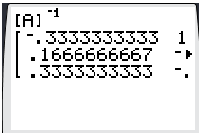Note that the calculator may return decimal versions of the fractions and will not show the entire matrix on its limited display. You will have to scroll to the right to confirm that $$A^{-1}$$ matches what you have already found. Once you have found $$A^{-1}$$ go ahead and store it as matrix $$B$$ so you do not need to type in the entries.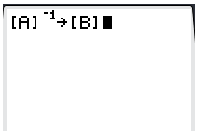$$A^{-1} \cdot A=B \cdot A$$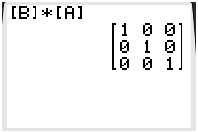$$A \cdot A^{-1}=A \cdot B$$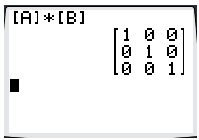$$A^{-1} \cdot\left[\begin{array}{c}96 \\ 36 \\ -12\end{array}\right]=B \cdot\left[\begin{array}{c}96 \\ 36 \\ -12\end{array}\right]=B \cdot C$$

You need to create matrix $$C=\left[\begin{array}{c}96 \\ 36 \\ -12\end{array}\right]$$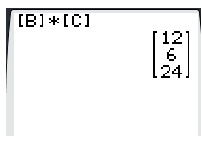Being able to effectively use a calculator should improve your understanding of matrices and allow you to check all the work you do by hand.

##### Example 5

The identity matrix happens to be its own inverse. Find another matrix that is its own inverse.

Helmert came up with a very clever matrix that happens to be its own inverse. Here are the $$2 \times 2$$ and the $$3 \times 3$$ versions.

$$\left[\begin{array}{cc}\frac{1}{\sqrt{2}} & \frac{1}{\sqrt{2}} \\ \frac{1}{\sqrt{2}} & -\frac{1}{\sqrt{2}}\end{array}\right],\left[\begin{array}{ccc}\frac{1}{\sqrt{3}} & \frac{1}{\sqrt{3}} & \frac{1}{\sqrt{3}} \\ \frac{1}{\sqrt{2}} & -\frac{1}{\sqrt{2}} & 0 \\ \frac{1}{\sqrt{6}} & \frac{1}{\sqrt{6}} & -\frac{2}{\sqrt{6}}\end{array}\right]$$

##### Review

Find the inverse of each of the following matrices, if possible. Make sure to do some by hand and some with your calculator.

1. $$\left[\begin{array}{ll}4 & 5 \\ 2 & 3\end{array}\right]$$

2. $$\left[\begin{array}{cc}-3 & 6 \\ 2 & 5\end{array}\right]$$

3. $$\left[\begin{array}{cc}-1 & 2 \\ 2 & 0\end{array}\right]$$

4. $$\left[\begin{array}{ll}1 & 6 \\ 0 & 1\end{array}\right]$$

5. $$\left[\begin{array}{cc}6 & 5 \\ 2 & -2\end{array}\right]$$

6. $$\left[\begin{array}{ll}4 & 2 \\ 6 & 3\end{array}\right]$$

7. $$\left[\begin{array}{ccc}-1 & 3 & -4 \\ 4 & 2 & 1 \\ 1 & 2 & 5\end{array}\right]$$

8. $$\left[\begin{array}{ccc}4 & 5 & 8 \\ 9 & 0 & 1 \\ 0 & 3 & -2\end{array}\right]$$

9. $$\left[\begin{array}{ccc}0 & 7 & -1 \\ 2 & -3 & 1 \\ 6 & 8 & 0\end{array}\right]$$

10. $$\left[\begin{array}{ccc}4 & 2 & -3 \\ 2 & 4 & 5 \\ 1 & 8 & 0\end{array}\right]$$

11. $$\left[\begin{array}{ccc}-2 & -6 & -12 \\ -1 & -5 & -2 \\ 2 & 3 & 4\end{array}\right]$$

12. $$\left[\begin{array}{ccc}-2 & 6 & 3 \\ 2 & 4 & 0 \\ -8 & 2 & 1\end{array}\right]$$

13. Show that Helmert's $$2 \times 2$$ matrix is its own inverse: $$\left[\begin{array}{ll}\frac{1}{\sqrt{2}} & \frac{1}{\sqrt{2}} \\ \frac{1}{\sqrt{2}} & -\frac{1}{\sqrt{2}}\end{array}\right]$$

14. Show that Helmert's $$3 \times 3$$ matrix is its own inverse: $$\left[\begin{array}{ccc}\frac{1}{\sqrt{3}} & \frac{1}{\sqrt{3}} & \frac{1}{\sqrt{3}} \\ \frac{1}{\sqrt{2}} & -\frac{1}{\sqrt{2}} & 0 \\ \frac{1}{\sqrt{6}} & \frac{1}{\sqrt{6}} & -\frac{2}{\sqrt{6}}\end{array}\right]$$

15. Non-square matrices sometimes have left inverses, where $$A^{-1} \cdot A=I$$, or right inverses, where $$A \cdot A^{-1}=I$$. Why can't non-square matrices have "regular" inverses?

8.9 Inverse Matrices is shared under a CC BY-NC license and was authored, remixed, and/or curated by LibreTexts.